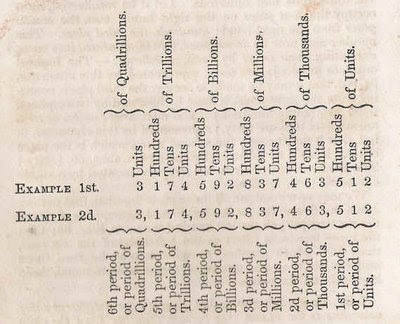## Wednesday, April 22, 2009

### Inflated Numbers

The word 'trillion' comes up with increasing regularity, and it seems that we are going to have to get used to finding the right words for larger and larger numbers.

In 1827, the textbook "Adam's New Arithmetic" (a pdf of the 1810 edition is available here) discussed numeration as far as the quadrillions - a real term, unlike gazillion.Thankfully, wikipedia takes us all the way to centillion.

The name for 10^x makes more sense if you consider n = x/3 - 1. So, for "octodecillion", which is 10^57, we look at 57/3 -1 = 18, which is octo (8) + deci (10). The "divide by three" occurs because each name gets three positions (e.g. millions, tens of millions, hundreds of millions). The "minus one" occurs since the naming scheme begins with millions, rather than thousands.